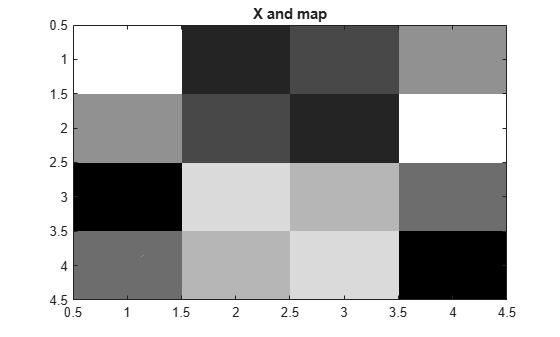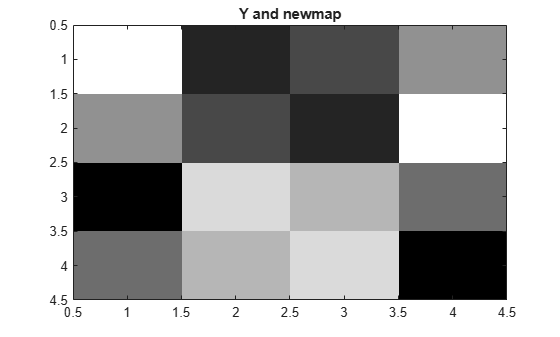# cmunique

Eliminate duplicate colors in colormap; convert grayscale or truecolor image to indexed image

## Syntax

``[Y,newmap] = cmunique(X,map)``
``[Y,newmap] = cmunique(RGB)``
``[Y,newmap] = cmunique(I)``

## Description

example

````[Y,newmap] = cmunique(X,map)` removes duplicate rows from the colormap `map` to produce a new colormap, `newmap`. The function also adjusts the indices in intensity image `X` to maintain correspondence between the indices and the colormap, and returns the result in `Y`. The image `Y` and associated colormap `newmap` produce the same image as `X` and `map` but with the smallest possible colormap.```
````[Y,newmap] = cmunique(RGB)` converts the truecolor image `RGB` to the indexed image `Y` and its associated colormap, `newmap`. The returned colormap is the smallest possible colormap for the image, containing one entry for each unique color in `RGB`. Note`newmap` might be very large, because the number of entries can be as many as the number of pixels in `RGB`. ```
````[Y,newmap] = cmunique(I)` converts the grayscale image `I` to an indexed image `Y` and its associated colormap, `newmap`. The returned colormap is the smallest possible colormap for the image, containing one entry for each unique intensity level in `I`.```

## Examples

collapse all

Use the `magic` function to define `X` as a 4-by-4 array that uses every value in the range between 1 and 16.

`X = magic(4);`

Use the `gray` function to create an eight-entry colormap. Then, concatenate the two eight-entry colormaps to create a colormap with 16 entries, `map`. In `map`, entries 9 through 16 are duplicates of entries 1 through 8.

```map = [gray(8); gray(8)]; size(map)```
```ans = 1×2 16 3 ```

Use `cmunique` to eliminate duplicate entries in the colormap.

```[Y, newmap] = cmunique(X, map); size(newmap)```
```ans = 1×2 8 3 ```

`cmunique` adjusts the values in the original image `X` so that `Y` and `newmap` produce the same image as `X` and `map`.

```figure image(X) colormap(map) title('X and map')``````figure image(Y) colormap(newmap) title('Y and newmap')```## Input Arguments

collapse all

Indexed image with duplicate colors, specified as an m-by-n matrix of integers.

Data Types: `double` | `uint8` | `uint16`

Colormap with duplicate colors associated with indexed image `X`, specified as a c1-by-3 matrix with values in the range [0, 1]. Each row of `map` is a three-element RGB triplet that specifies the red, green, and blue components of a single color of the colormap.

Data Types: `double`

RGB image, specified as an m-by-n-by-3 array of nonnegative numbers.

Data Types: `double` | `uint8` | `uint16`

Grayscale image, specified as an m-by-n numeric matrix.

Data Types: `double` | `uint8` | `uint16`

## Output Arguments

collapse all

Indexed image with unique colors, returned as an m-by-n matrix of integers. If the length of `newmap` is less than or equal to 256, then the output image is of class `uint8`. Otherwise, the output image is of class `double`.

Data Types: `double` | `uint8`

Colormap with unique colors associated with the output indexed image `Y`, returned as a c2-by-3 matrix with values in the range [0, 1]. Each row of `newmap` is a three-element RGB triplet that specifies the red, green, and blue components of a single color of the colormap.

Data Types: `double`